Courses

# Engineering Mathematics & General Aptitude - MCQ Test 1

## 15 Questions MCQ Test Mock Tests Electronics & Communication Engineering GATE 2020 | Engineering Mathematics & General Aptitude - MCQ Test 1

Description
This mock test of Engineering Mathematics & General Aptitude - MCQ Test 1 for Electronics and Communication Engineering (ECE) helps you for every Electronics and Communication Engineering (ECE) entrance exam. This contains 15 Multiple Choice Questions for Electronics and Communication Engineering (ECE) Engineering Mathematics & General Aptitude - MCQ Test 1 (mcq) to study with solutions a complete question bank. The solved questions answers in this Engineering Mathematics & General Aptitude - MCQ Test 1 quiz give you a good mix of easy questions and tough questions. Electronics and Communication Engineering (ECE) students definitely take this Engineering Mathematics & General Aptitude - MCQ Test 1 exercise for a better result in the exam. You can find other Engineering Mathematics & General Aptitude - MCQ Test 1 extra questions, long questions & short questions for Electronics and Communication Engineering (ECE) on EduRev as well by searching above.
QUESTION: 1

### The cost price of 20 articles is the same as the selling price of x articles. If the profit is 25%, then the value of'?; is:

Solution: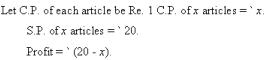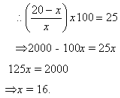QUESTION: 2

### A father said to his son, "I was as old as you are at the present at the time of your birth". If the father’s age is 38 years now, the son’s age five years back was  ____________years

Solution: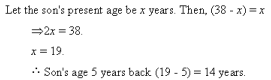QUESTION: 3

### In how many different ways can the letters of the word 'LEADING' be arranged in such a way that the vowels always come together?

Solution:

The word 'LEADING' has 7 different letters.
When the vowels EAI sire always together, they can be supposed to form one letter. Then, we have to arrange the letters LNDG (EAI).
Now, 5 (4 + 1 = 5) letters can be arranged in 5! = 120 ways.
The vowels (EAI) can be arranged among themselves in 3! = 6 ways.
Required number of ways = (120 x 6) = 720.

QUESTION: 4

If a person walks at 14 km/hr instead of 10 km/hr, he would have walked 20 km more. The actual distance travelled by him is  ______________km

Solution: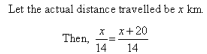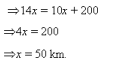QUESTION: 5

Today is Monday. After 61 days, it will be:

Solution:

Each day of the week is repeated after 7 days. So, after 63 days, it will be Monday.

After 61 days, it will be Saturday.

QUESTION: 6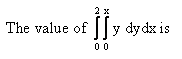Solution: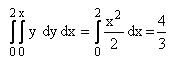QUESTION: 7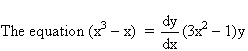mth initial condition y(1) = 0 has

Solution:
QUESTION: 8

The probability of at least one of A and B occurring is 3/5 and that of A and B occurring together is 3/10 then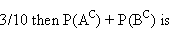Solution: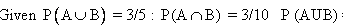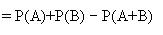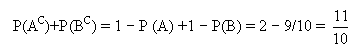QUESTION: 9

The order of convergence of the iterative formulaSolution:

QUESTION: 10

If f(x,y) = xy+(x-y) then the saddle point is

Solution: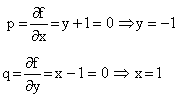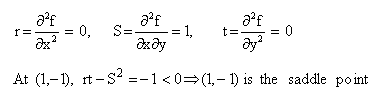QUESTION: 11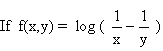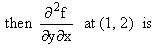Solution: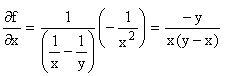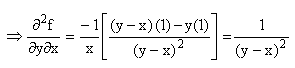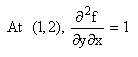QUESTION: 12

Direction: Which of the following options is the closest in the meaning to the word below?

FLAMBOYANT

Solution:

DEFINITION: brightly colored, florid
SYNONYMS: showy, ostentatious, flashy, gaudy, bright, colorful, brilliant, exciting

QUESTION: 13

Direction: The question  below consist of a pair of related words followed by four pairs of words. Select the pair that best expresses the relation in the original pair:

RIND : MELON ::

Solution:

(PART AND THE WHOLE) Rind is the outer cover of a melon; skin is the outer cover of an apple. (B)

QUESTION: 14

Direction: Choose the most appropriate word(s) from the options given below to complete the following sentence

In spite of the increasing _________  of their opinions, the group knew they had to arrive at a consensus so that theaward could be presented.

Solution:

The phrase ‘in spite of at the beginning of the sentence logically implies that there is a contradiction of ideas within the sentence. You can guess from the sentence that the .group mentioned in it has been formed in order to select a person who has to be given an award for some specific performance.    The second part says
that the group knew that they had to arrive at a consensus (meaning unanimity or agreement) for the award to be presented. The use of the phrase ‘in spite of in the beginning of the sentence means that the present opinions of the members of the group constitute a status which is the opposite of ‘ consensus’.The missing word should therefore mean ‘disagreement’, ‘divergence’, ‘controversy’ etc.
Among the choices, it is ‘polarity’ which means ‘divergence’ and is the answer.

QUESTION: 15

Direction: Choose the word from the options given below that is most nearly opposite in meaning to the given word.

PROFUSE :

Solution:

Profuse means abundant; lavish; luxuriant. Its opposite is scanty, meaning little, meager, scarce.